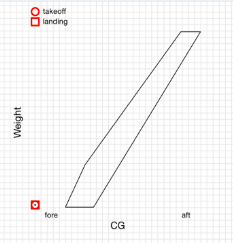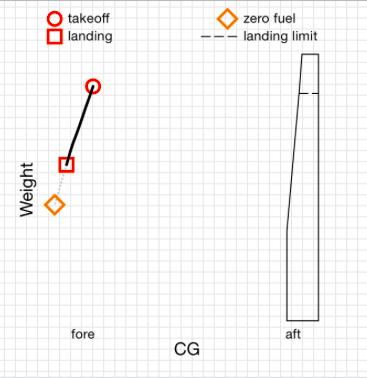# Why can't I get my CG into the CG envelope at all?

Is your CG Envelope Slanted as pictured below and/or the CG not able to come into the envelope?Image 1. Probable CG Envelope data problem.

If so, please check that the numbers entered to define the CG Envelope are Arms and not Divided Moments.  Some Pilot's Operating Handbooks (POH) have a CG Envelope that displays a CG envelope with a Moment number that has been divided by 100 or 1000.  This will make the numbers look potentially like an Arm.

If you find this is the case, you can take the Moment number listed and multiply it by the number the document claims the Moment was divided by.  Once you have done that, divide that number by the corresponding weight to get the Arm.

An example is as follows.  If a Moment was divided by 1000, take the displayed number (in this example 23.5) and multiply by 1000.  Next, divide that number by the corresponding weight (in this example 1650 lbs).  Formula:  (23.5x1000)/1650 = 14.24  -OR-  Total Moment/Weight=Arm